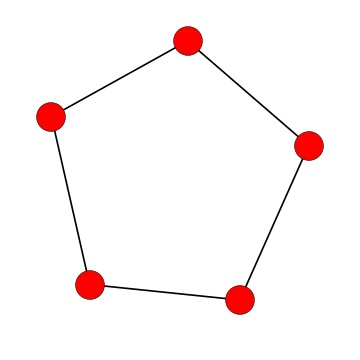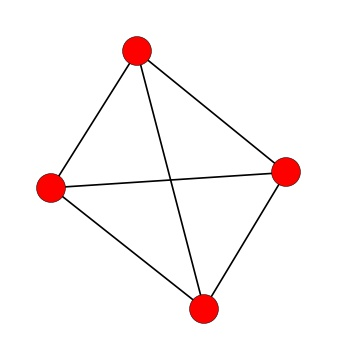Probability

# Planar GraphsIs this graph planar?Is this graph planar?

Suppose a graph $G$ is not planar. If we add an edge to $G$ to connect two previously disconnected nodes to create the graph $G'$, is $G'$ planar?

Suppose that there are three houses $A, B, C$ and three utilities 1, 2, and 3 each of which needs to be connected by a wire to all three houses. Assuming the utilities and the houses are all points (nodes), is there a way to position them and the wires (edges) such that no two wires overlap?

Note: this is the same as asking whether $K_{3,3}$ is a planar graph.

Suppose $G$ is a planar graph. If we create $G'$ by adding a single edge to $G$, is $G'$ planar?

×